# RANK Function

Returns the statistical rank of a given value within an array of values

## What is the RANK Function?

The RANK Function is categorized as an Excel Statistical function. The function returns the statistical rank of a given value within a supplied array of values. Thus, it determines the position of a specific value in an array.

### Formula

=RANK(number,ref,[order])

The RANK function uses the following arguments:

1. Number (required argument) – This is the value for which we need to find the rank.
2. Ref (required argument) – Can be a list of, or an array of, or reference to, numbers.
3. Order (optional argument) – This is a number that specifies how the ranking will be done (ascending or descending order).
• 0 – is used for descending order
• 1 – is used for ascending order
• If we omit the argument, it will take a default value of 0 (descending order). It will take any non-zero value as the value 1 (ascending order).

Before we proceed, we need to know that the RANK function has been replaced by RANK.EQ and RANK.AVG. To enable backward compatibility, RANK still works in Excel 2016 (latest version), but it may not be available in the future. If you type this function in Excel 2016, it will show a yellow triangle with an exclamation point.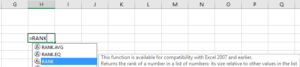### How to use the RANK Function in Excel?

As a worksheet function, RANK can be entered as part of a formula in a cell of a worksheet. To understand the uses of the function, let’s consider a few examples:

#### Example 1

Assume we are given a list of employees with their respective medical reimbursement expenses. We wish to rank them according to total expenditure.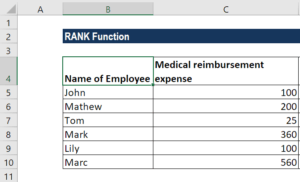To rank in descending order, we will use the formula =RANK(B2,(\$C\$5:\$C\$10),0), as shown below: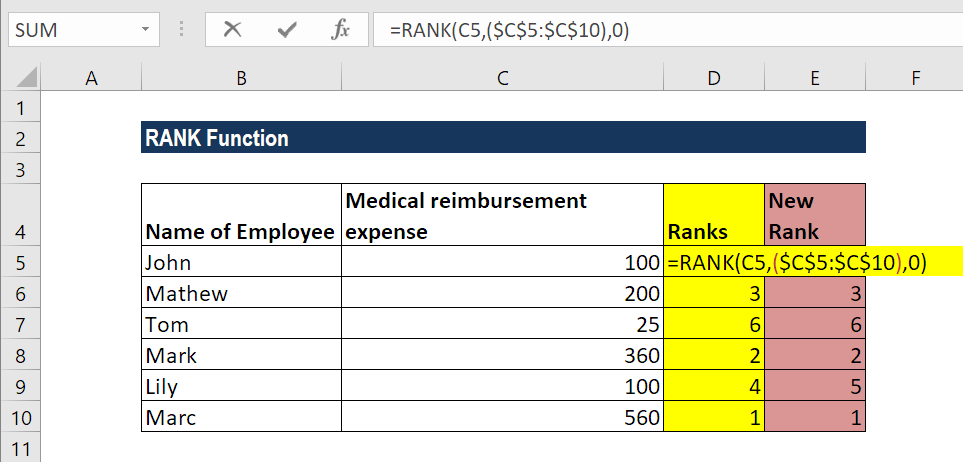The result we get is shown below: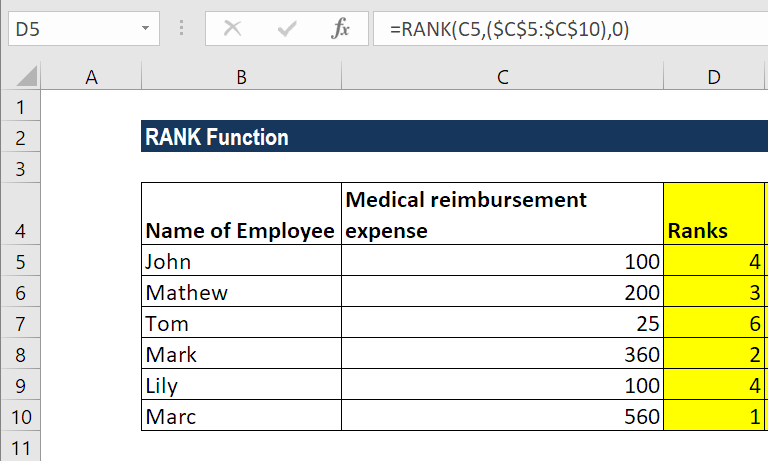As seen above, the RANK function gives duplicate numbers the same rank. However, the presence of duplicate numbers affects the ranks of subsequent numbers. For example, as shown above, in a list of integers sorted in ascending order, the number 100 appears twice with a rank of 4. The next value (25) will be ranked 6 (no number will be ranked 5).

If we want unique ranks, we can use the formula:

=RANK(C5,\$C\$5:C\$10,0)+COUNTIF(\$C\$5:C5,C5)-1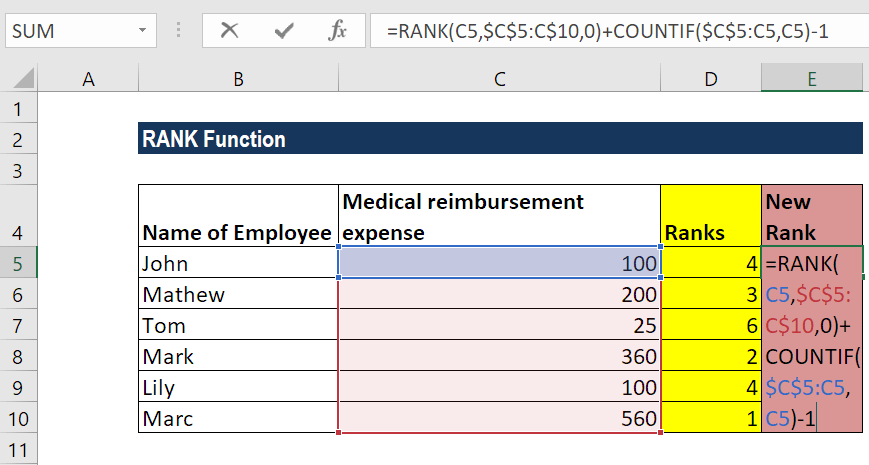We will get the results below: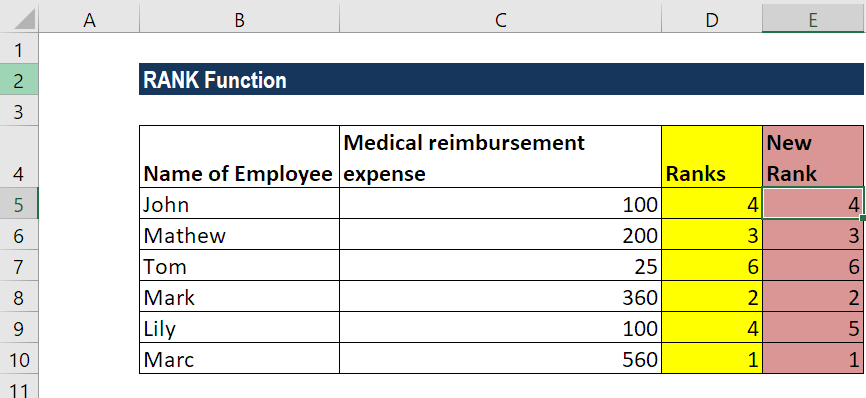For ascending order, the formula would be:

=RANK.EQ(C5,\$C\$5:C\$10,1)+COUNTIF(\$C\$5:C5,C5)-1

In both formulas, it’s the COUNTIF function that does the trick. We used COUNTIF to find out the number of times the ranked number occurred. In the COUNTIF formula, the range consists of a single cell (\$C\$5:C5). As we locked only the first reference (\$C\$5), the last relative reference (C5) changes based on the row where the formula is copied. Thus, for row 7, the range expands to \$C\$5:C10, and the value in C10 is compared to each of the above cells.

Thus, for all unique values and first occurrences of duplicate values, COUNTIF returns 1; and we subtract 1 at the end of the formula to restore the original rank.

For ranks occurring the second time, COUNTIF returns 2. By subtracting 1, we increased the rank by 1 point, thus preventing duplicates. If there happen to be 3+ occurrences of the same value, COUNTIF()-1 would add 2 to their ranking, and so on.

### Things to remember about the RANK Function

1. #N/A! error – Occurs when the given number is not present in the supplied reference. Also, the RANK function does not recognize text representations of numbers as numeric values, so we will also get the #N/A error if the values in the supplied ref array are text values.
2. If we provide logical values, we will get the #VALUE! error.

Thanks for reading CFI’s guide to important Excel functions! By taking the time to learn and master these functions, you’ll significantly speed up your financial analysis. To learn more, check out these additional CFI resources:

• Excel Functions for Finance